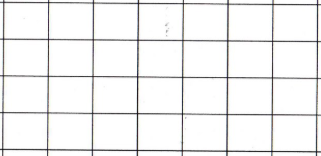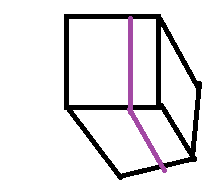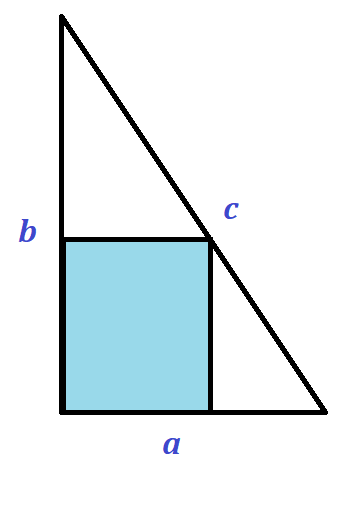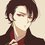# Some bizarre questions that need urgent attention?!!

Can anyone help with these easy, medium and hard questions?

(1) How many ways are there of placing a single $3 \times 1$ rectangle on this grid so that it completely covers 3 grid squares?(2) A number is a palindrome if it reads the same as forwards as backwards. The number 131131 is a palindrome; also its first pair of digits, middle and the last pair are prime numbers. How many such 6-digit palindromes are there?

(3) I have a large number of toy soldiers, which I can arrange into a rectangle array consisting of rows and a number of columns. I notice that if I remove 100 toy soldiers, then I can arrange the remaining ones into a rectangle with 5 fewer rows and 5 more columns.

How many toy soldiers would I have to remove from the original configuration to be able to arrange the remaining ones into a rectangular array with 11 fewer rows and 11 more columns?

(4) How many 3 digit numbers are there such that it is equal to the sum of its digits multiplied by 13?

(5) 1 Googol is $G = 10^{100}$ whereas 1 Googleplex is $10^G$. Let $n$ be the largest whole number for which $n^n < 10^G.$ How many digits does $n$ have?

(6) There is a square tabletop filled with small square-shaped tiles. The sum of the squares that form the 2 diagonals is 25. Find the area of the tabletop.

(7) I have a cube which has a surface area of $X$. I slice it into 2 to get 2 (different or same) prisms. (The diagram shows how it has been cut). If one of the prism's surface area is $\dfrac{1}{2}$ of $X$, then find the surface area of the other prism in relation to $X$.(8) This is a right-angled triangle. Find the ratio between the unshaded region to the shaded region using the letters in all cases. $a, b,c$. Note the blue colour shaded is a square.(9) A quadrilateral has two parallel sides measuring 25 cm and 37 cm. What is the distance in centimetres, between the midpoints of the diagonals?

That's all for today, folks!

I also wanted to make note of Q5; I myself tried it and came till here; if anyone can help so tell me in the comments.

We know:

$G = 10^{100}$ and 1 Googlepex is = $10^G = 10^{10^{100}}$ .

To find the number of digits of $n$, we have to find an exponential representation of $n$. Then we can use the theorem:

For any positive integer $n$, the number of digits in $n$ is $\left\lfloor \log_{10} n \right\rfloor + 1$. (It can be found on this wiki )

So, I tried to use the inequality: $\large n^n < 10^{10^{100}}$

First I converted into an equation: $n^n = 10^{10^{100}}$ and then I didn't understand how to subject $n$.

These are what I attempted: $\log_n 10^{10^{100}} = n \implies G \log_n 10 = n$

I might be adding some more problems in this note this week only so please be updated. I hope to get answers with extremely clear language and makes notes of all theorems used in solving with each method presented carefully and neatly. Please make use of Latex in your answers. I thank everyone who tried.Note by Syed Hamza Khalid
2 years, 10 months ago

This discussion board is a place to discuss our Daily Challenges and the math and science related to those challenges. Explanations are more than just a solution — they should explain the steps and thinking strategies that you used to obtain the solution. Comments should further the discussion of math and science.

When posting on Brilliant:

• Use the emojis to react to an explanation, whether you're congratulating a job well done , or just really confused .
• Ask specific questions about the challenge or the steps in somebody's explanation. Well-posed questions can add a lot to the discussion, but posting "I don't understand!" doesn't help anyone.
• Try to contribute something new to the discussion, whether it is an extension, generalization or other idea related to the challenge.

MarkdownAppears as
*italics* or _italics_ italics
**bold** or __bold__ bold
- bulleted- list
• bulleted
• list
1. numbered2. list
1. numbered
2. list
Note: you must add a full line of space before and after lists for them to show up correctly
paragraph 1paragraph 2

paragraph 1

paragraph 2

[example link](https://brilliant.org)example link
> This is a quote
This is a quote
    # I indented these lines
# 4 spaces, and now they show
# up as a code block.

print "hello world"
# I indented these lines
# 4 spaces, and now they show
# up as a code block.

print "hello world"
MathAppears as
Remember to wrap math in $$ ... $$ or $ ... $ to ensure proper formatting.
2 \times 3 $2 \times 3$
2^{34} $2^{34}$
a_{i-1} $a_{i-1}$
\frac{2}{3} $\frac{2}{3}$
\sqrt{2} $\sqrt{2}$
\sum_{i=1}^3 $\sum_{i=1}^3$
\sin \theta $\sin \theta$
\boxed{123} $\boxed{123}$

Sort by:

Q.1 7(4-2)+4(7-2)=34

- 2 years, 10 months ago

How? Is there some kind of theorem, formula or something?

- 2 years, 10 months ago

You can try to place the 1x3 on the 4x7 grid, and you will know what the expression means.

- 2 years, 10 months ago

@X X Actually, I didn't get it yet. can u give a slightly detailed answer, please?

- 2 years, 10 months ago

- 2 years, 10 months ago

- 2 years, 10 months ago

Consider it as two kinds. One is to put the 1x3 horizontally, and the other is to put it vertically.

- 2 years, 10 months ago

@X X Put it horizontally makes it 20 possibilities, and vertically makes it 14 possibilities.

- 2 years, 10 months ago

@X X yeah got it thanks

- 2 years, 10 months ago

Can u help for other problems?

- 2 years, 10 months ago

Q.5 $(10^a)^{(10^a)}=10^{a\times10^a}$, so $a\times10^a$ need to be close to $10^{100}$. We have $98\times10^{98}<10^{100}<99\times10^{99}$ , so $10^{98}

- 2 years, 10 months ago

So what's the final answer? You have not exactly responded to the question { we have to find the number of digits in $n$}

- 2 years, 10 months ago

If $10^{98}, then it is easy to know the number of digits in $n$

- 2 years, 10 months ago

@X X Yep I got that!

- 2 years, 10 months ago

@X X Is the answer between 99 and 100? However, for this question, only integers are allowed!

- 2 years, 10 months ago

Actually, the answer will simply come out to be 99!!!

- 2 years, 10 months ago

Can u give some explanation, why? when? how? to use something?

- 2 years, 10 months ago

I let $n=10^a$ because if I find the $a$ then i will know the number of digits of $n$.

- 2 years, 10 months ago

@X X Oh okay you should have mentioned that because I was shocked to see $a$ [I had no idea what it was]

- 2 years, 10 months ago

Sorry, I forgot to say that. Thanks for pointing out!

- 2 years, 10 months ago

@X X Welcome

- 2 years, 10 months ago

7.Let the area of each face of the cube be $x$, so $X=6x$.

If we slice it into two, then the surface area will increase by $2x$, so it will become $8x$.

One of the prism has surface area of $3x$, because it has half the surface area of the original cube.

So, the other prism has surface area of $8x-3x=5x$

- 2 years, 10 months ago

Q.4 The answer is 3 such numbers....viz 117,156 and 195

- 2 years, 10 months ago

How did u find it

- 2 years, 10 months ago

Well Let the number be abc.......
Now, according to the question,
87a = 3b +12c i.e.
29a = b +4c
Now observe that a can only be equal to one..........I think you can solve it now

- 2 years, 10 months ago

How do you know that these are the only no.s

- 2 years, 10 months ago

Q.2

let $abcdef$ represent a six-digit number and each letter represent one digit. For $abcdef$ to be a palindrome, it must be the same forwards and backwards. Therefore, as long as $a=f$, $b=e$, and $c=d$, there are $9\times 10\times 10=900$ six digit palindromes.

- 2 years, 10 months ago

how? in addition to that it should be prime?

- 2 years, 10 months ago

We are taking here all the possibilities.

- 2 years, 10 months ago

But that is not the question...

- 2 years, 10 months ago

8.Let the side length of the square be $x$. There are some triangle that are similar(Sorry, I'm lazy to draw a picture for this), so $(b-x):x=b:a, ab-ax=bx,x=\frac{ab}{a+b}$.

The area of the square is $\frac{a^2b^2}{(a+b)^2}$, and the area of the big triangle is $\frac{ab}2$.

So, the ratio between the square and the triangle is $2ab:(a+b)^2$,

and the ratio between the colored region and the white region is $2ab:(a^2+b^2+2ab-2ab)=2ab:(a^2+b^2)$.

- 2 years, 10 months ago

Wait,how about $c$?You missed $c$!

- 2 years, 10 months ago

Oh, you probably means $2ab:c^2$.

- 2 years, 10 months ago

Thanks for your effort. I had the AMC and I screwed up some easy questions :(

I will try again next year and hope for a score > 100.

- 2 years, 10 months ago

Q.3 $ab-100=(a+5)(b-5),15=a-b$, so $ab-(a+11)(b-11)=11a-11b+121=11\times15+121=286$

- 2 years, 10 months ago

- 2 years, 10 months ago

Let there are a columns and b rows. The other parts are algebra.

- 2 years, 10 months ago

@X X Thanks! Got it; but at the end what did you do? 121 = 44? I don't get that.

Shouldn't it be like $\implies 11a - 11b + 121 = 11(a - b) +121 = (11 \times 15) +121 = \boxed{ 286 }$

- 2 years, 10 months ago

Sorry, two typos(such a big mistake!).

- 2 years, 10 months ago

@X X Its okay; we all are humans! Can u help me for the Q1 method with reasoning?

- 2 years, 10 months ago